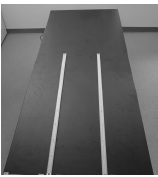# Investigation 3 – Lab### MINDSET

This Investigation is designed to:

• allow you to demonstrate that the speed of sound is different in different types of matter,
• allow you to demonstrate that in different types of matter, the frequency remains constant but the wavelength changes,
• allow you to use the equation relating the speed of sound to the frequency and the wavelength, and
• allow you to demonstrate the reciprocal relationship between the frequency and the wavelength of sound.

### BE PREPARED

Student Preparation for the Investigation includes gathering the following materials.

Note: The materials are listed in your SDR. They are also listed below for your reference.

• (1) 50ml beaker
• (1) 50ml beaker filled with water
• (1) iron electrode
• (2) meter sticks
• (1) calculator
• (1) one meter length of string
• (1) roll of masking tape
• (1) lab marker

Direct one student from each lab group to collect the materials listed in their SDRs.

### INVESTIGATE

• You will model the speed of sound in water and iron.
• You will also model the speed of sound in air.
• You will perform three Trials during this Investigation. In each Trial, you will calculate the speed of sound in a different type of matter.
• You will analyze your data and identify which of the three types of matter is air, water, or iron.
• Read the Background before Trial 1 to remind yourself of the relationship between speed, frequency, and wavelength.
• Reflect on the PreLab video as you move through the procedural steps.
• During the Experiment, every procedural step is important. If one step is skipped, data can become invalid. To help you keep on track, read each step thoroughly, complete the step, then check it off (Read it – Do it – Check it).
• Complete all of the procedural steps in your SDR.

Note: The procedural steps are listed below for your reference.

The Experiment includes the following:

1. In this Investigation, you will model how different types of matter affect the speed of sound in each type of matter. You will assume that the pressure wave in each type of matter has a specific frequency but that the wavelength of the pressure wave in each type of matter is different. You will then calculate the speed of sound in each type of matter.
2. Place the two meter sticks on the table so that the width of the table is divided into three equal sections.1. You will model the speed of sound in three types of matter, air, water, and iron. Observe the contents of the empty beaker, the water in the second beaker, and the iron electrode in the third beaker and answer the following questions.
1. Describe the contents of the empty beaker.
1. Describe the water filling the second beaker.
1. Describe the iron electrode.
1. For each of the three types of matter, refer to Table A for the frequency and wavelength of the pressure waves in each type of matter. Notice that the frequency of the sound wave in each type of matter is 4,000 Hertz but the wavelengths are different.

Trial 1:

1. In this Trial, you will model the sound waves that travel through the first type of matter in the beaker.
2. Refer to the wavelength in Table A. Use the meter sticks to measure the wavelength of the sound wave starting at the end of the meter sticks.
3. Cut a piece of string equal to the wavelength. Tape one end at the end of the table. Arrange the string on the table to represent the sound wave of the correct wavelength. Tape the other end of the string to the table using a second piece of masking tape.
4. Use the equation relating speed, frequency, and wavelength to calculate the speed of sound in the first type of matter. Use the space below for your calculations. Record the speed in Table A.

Trial 2:

1. In this Trial, you will model the sound waves that travel through the second type of matter in the beaker.
2. Refer to the wavelength in Table A. Use the meter sticks to measure the wavelength of the sound wave starting at the end of the meter sticks.
3. Cut a piece of string equal to the wavelength. Tape one end at the end of the table. Arrange the string on the table to represent the sound wave of the correct wavelength. Tape the other end of the string to the table using a second piece of masking tape.
4. Use the equation relating speed, frequency, and wavelength to calculate the speed of sound in the second type of matter. Use the space below for your calculations. Record the speed in Table A.

Trial 3:

1. In this Trial, you will model the sound waves that travel through the third type of matter.
2. Refer to the wavelength in Table A. Use the meter sticks to measure the wavelength of the sound wave starting at the end of the meter sticks.
3. Cut a piece of string equal to the wavelength. Tape one end at the end of the table. Arrange the string on the table to represent the sound wave of the correct wavelength. Tape the other end of the string to the table using a second piece of masking tape.
4. Use the equation relating speed, frequency, and wavelength to calculate the speed of sound in the third type of matter. Use the space below for your calculations. Record the speed in Table A.

### CLEAN UP

Be sure to clean up your lab bench when you have finished your experiments.